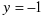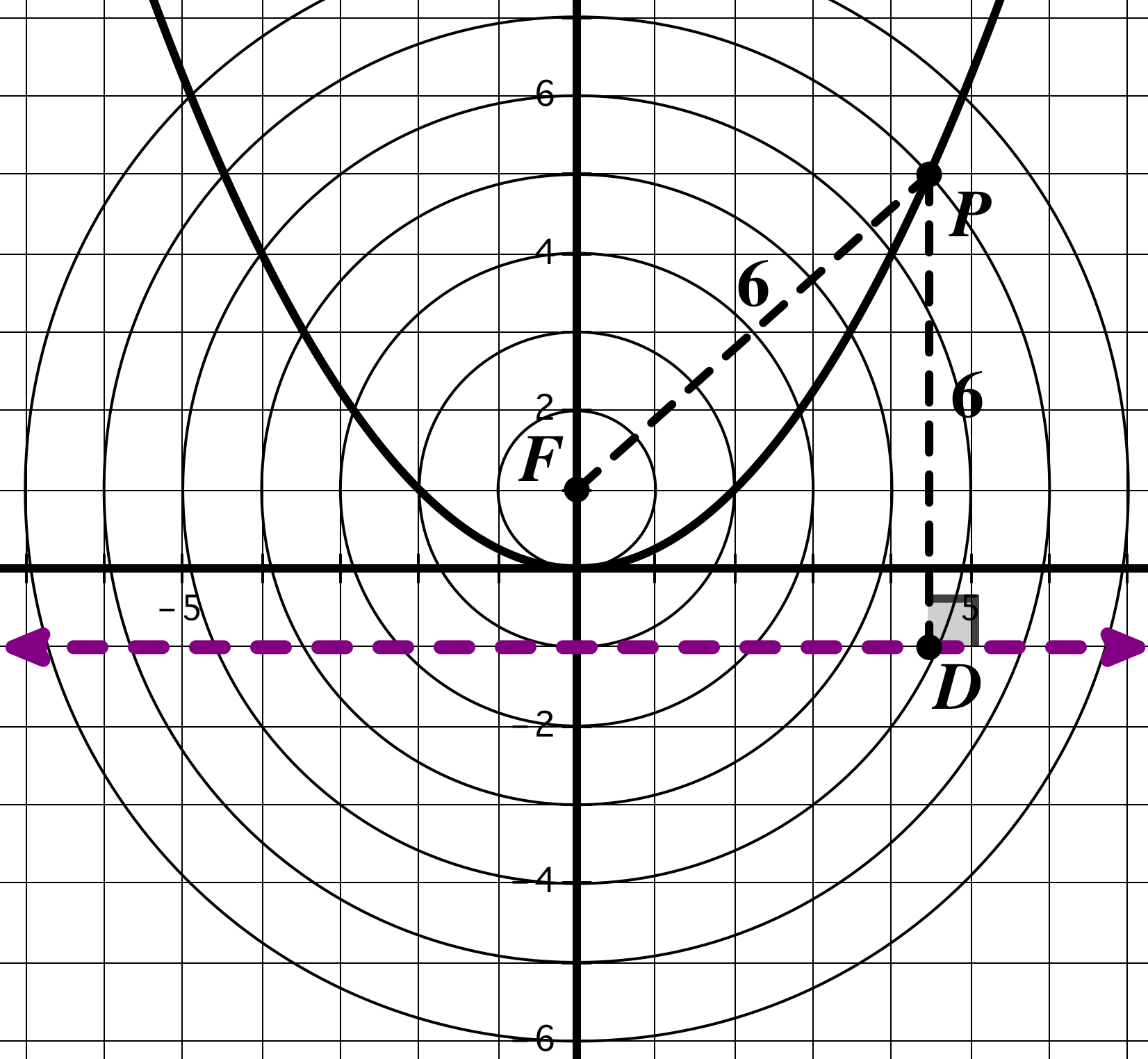# whose distance from the focus (F) equals its distance from the directrix

 Page 1/3 Date conversion 28.06.2018 Size 2.7 Mb.
1   2   3

Honors Math 2 Name:

Date:

Geometric (Locus) Definition of a Parabola
A parabola is a locus defined in terms of a fixed point, called the focus, and a fixed line, called the directrix. A parabola is the set of all points P(x, y) whose distance from the focus (F) equals its distance from the directrix. In other words, PF = PD (where D is the point on the directrix closest to

P – so PD is perpendicular to the directrix).

 The figure shows a parabola with its focus at (0, 1) and a directrix of. Equally spaced concentric circles with their center at the parabola’s focus enable you to measure distances from the focus. Equally spaced horizontal lines parallel to the parabola’s directrix enable you to measure vertical distances from the directrix. This type of graph paper is called focus-directrix graph paper. Notice that P is the point of intersection of the circle centered at (0, 1) with a radius of 6 and the horizontal line 6 units above the directrix. Thus, P is equidistant from the focus and directrix. Examine the figure and note that all points on the parabola are equidistant from the focus and the directrix.1   2   3